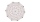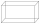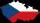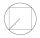# Triangle + rectangle - math problems

#### Number of problems found: 79

• Wall and body diagonalsCalculate the lengths of the wall and body diagonals of the cuboid with edge dimensions of 0.5 m, 1 m, and 2 m
• Area of a rectangleCalculate a rectangle area with a diagonal of u = 12.5cm and a width of b = 3.5cm. Use the Pythagorean theorem.
• Ratio of sidesCalculate the area of a circle with the same circumference as the circumference of the rectangle inscribed with a circle with a radius of r 9 cm so that its sides are in ratio 2 to 7.
• Triangular prismCalculate a triangular prism if it has a rectangular triangle base with a = 4cm and hypotenuse c = 50mm and height of the prism is 0.12 dm.
• CincinnatiA map is placed on a coordinate grid. Cincinnati located at (5,4) and San Diego is located at (-10, -3). How far apart is Cincinnati from San Diego on the map? Round to the nearest tenth.
• RectangleThere is a rectangle with a length of 12 cm and a diagonal 8 cm longer than the width. Calculate the area of a rectangle.
• Rectangular fieldA rectangular field has a diagonal of length 169m. If the length and width are in the ratio 12:5. Find the dimensions of the field, the perimeter of the field and the area of the field.
• Cuboidal roomLength of cuboidal room is 2m breadth of cuboidal room is 3m and height is 6m find the length of the longest rod that can be fitted in the room
• Axial cut of a rectangleCalculate the volume and surface of the cylinder whose axial cut is a rectangle 15 cm wide with a diagonal of 25 cm long.
• Rectangle JANOThe rectangle has side lengths | JA | = 16cm and | AN | = 12cm. Point S is the center of the JO side and point T is the center of the JA side. Calculate the perimeter of the pentagon in cm.
• Rectangle diagonalThe rectangle, one side of which is 5 cm long, is divided by a 13 cm diagonal into two triangles. Calculate the area of one of these triangles in cm2.
• Rectangular gardenThe sides of the rectangular garden are in ratio 1: 2. The diagonal has a length of 20 meters. Calculate the area and perimeter of the garden.
• Inscribed rectangleWhat is the perimeter of a rectangle that is inscribed in a circle whose diameter is 5 dm long? Answer: 14 dm
• Angle of diagonalThe angle between the body diagonal of a regular quadrilateral and its base is 60°. The edge of the base has a length of 10cm. Calculate the body volume.
• CZ flagWhat percentage of the Czech flag is made up of blue, white and red textiles?
• The Scout TentThe Scout Tent has a rectangular wooden underlay with dimensions of 220 cm and 150 cm. How much canvas is needed for a 170 cm high pyramid roof?
• Diagonal to areaCalculate the area of a rectangle in which the length of the diagonal is 10 cm.
• Find theFind the length of the side of the square ABCD, which is described by a circle k with a radius of 10 cm.
• Tv screenThe length of its diagonal gives the size of a tv screen. If the dimension of a tv screen is 16 inches by 14 inches, what is the TV screen size?
• The sidesThe sides of the rectangle are in a ratio of 3: 5 and its circumference measures 72 cm. Calculate: a) the size of both sides of the rectangle b) the area of the rectangle c) the length of the diagonals

Do you have an exciting math question or word problem that you can't solve? Ask a question or post a math problem, and we can try to solve it.

We will send a solution to your e-mail address. Solved examples are also published here. Please enter the e-mail correctly and check whether you don't have a full mailbox.

Please do not submit problems from current active competitions such as Mathematical Olympiad, correspondence seminars etc...